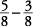As you review adding and subtracting fractions, notice the steps that are the same for both operations.

To add fractions, you must have a common denominator. Fractions that have common denominators are called like fractions. Fractions that have different denominators are called unlike fractions. To add like fractions, simply add the numerators and keep the same (or like) denominator.

AddTo add unlike fractions, first change all denominators to their lowest common denominator (LCD), also called the lowest common multiple of the denominator, the lowest number that can be divided evenly by all denominators in the problem. The numerators may need to be changed to make sure that the fractions are still equivalent to the originals. When you have all the denominators the same, you may add the numerators and keep the same denominator.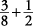Change thetobecause the 8 is the lowest common denominator; then add the numerators 3 and 4 to get.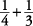Change both fractions to get the lowest common denominator of 12, and then add the numerators to get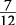.Note: Fractions may be added across as well.

Add.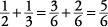To subtract fractions, the same rule as in adding fractions applies (find the LCD), except that you subtract the numerators.

Subtract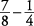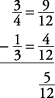Again, a subtraction problem may be done across as well as down.

Subtract